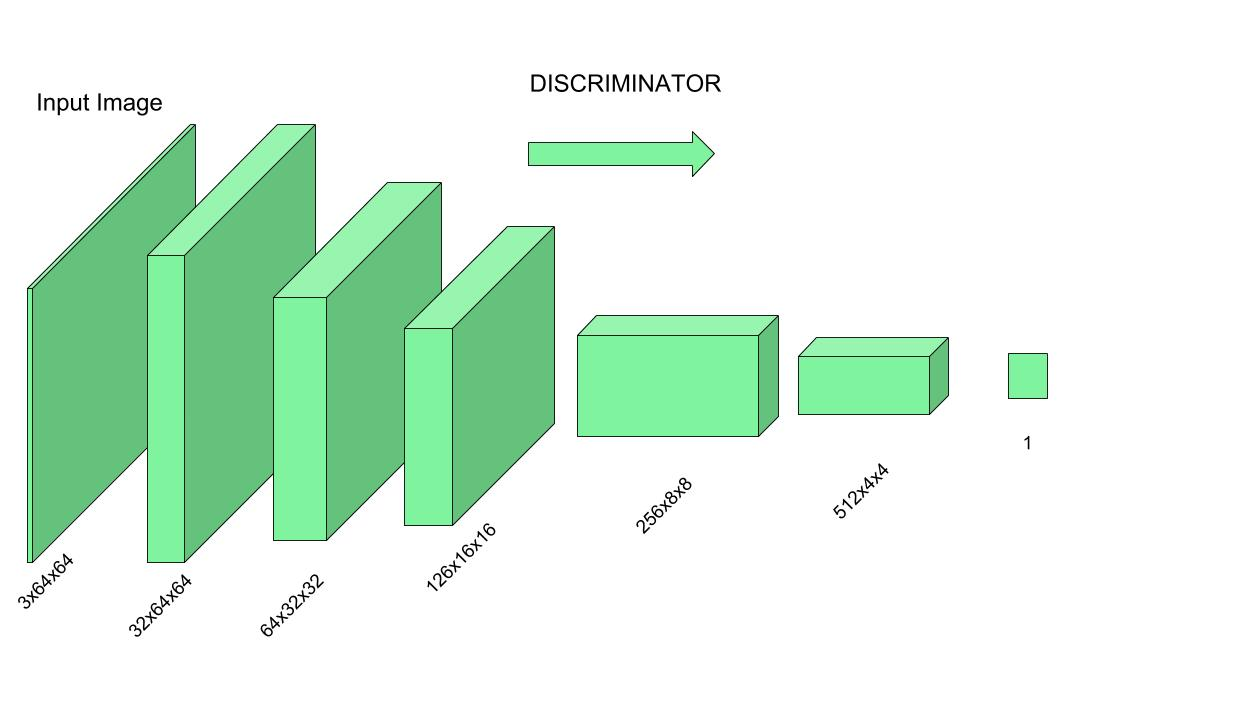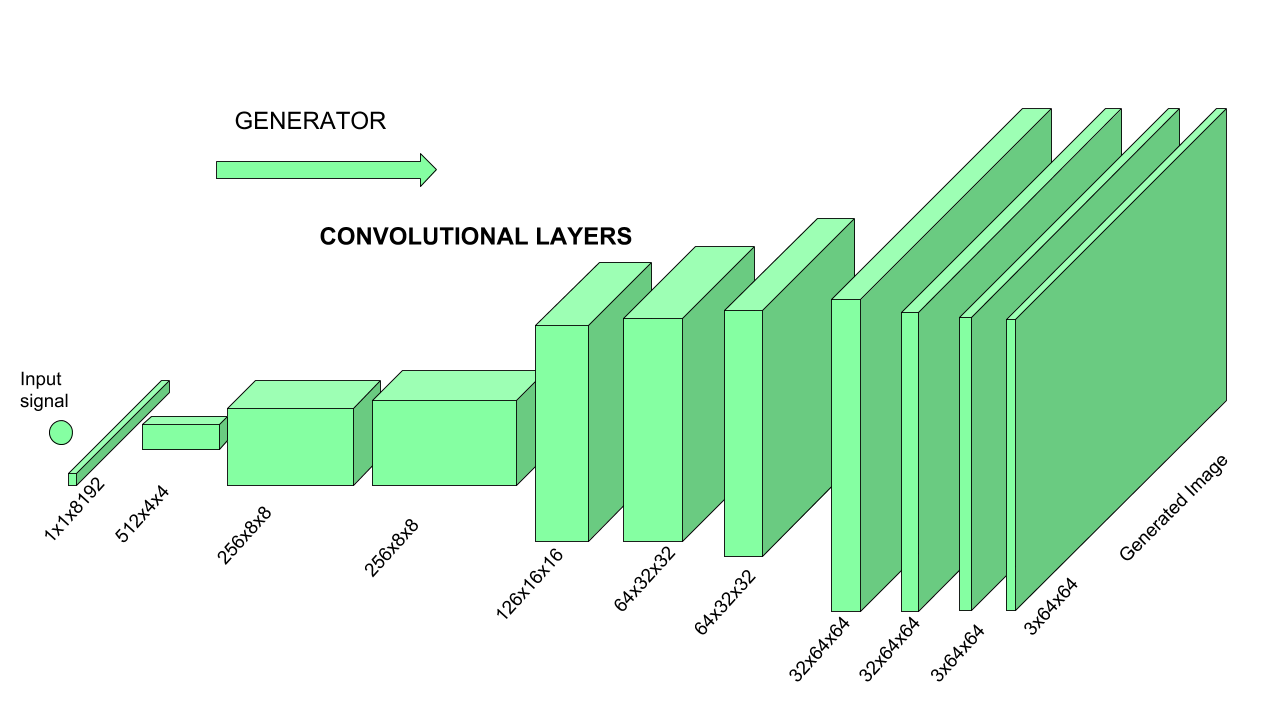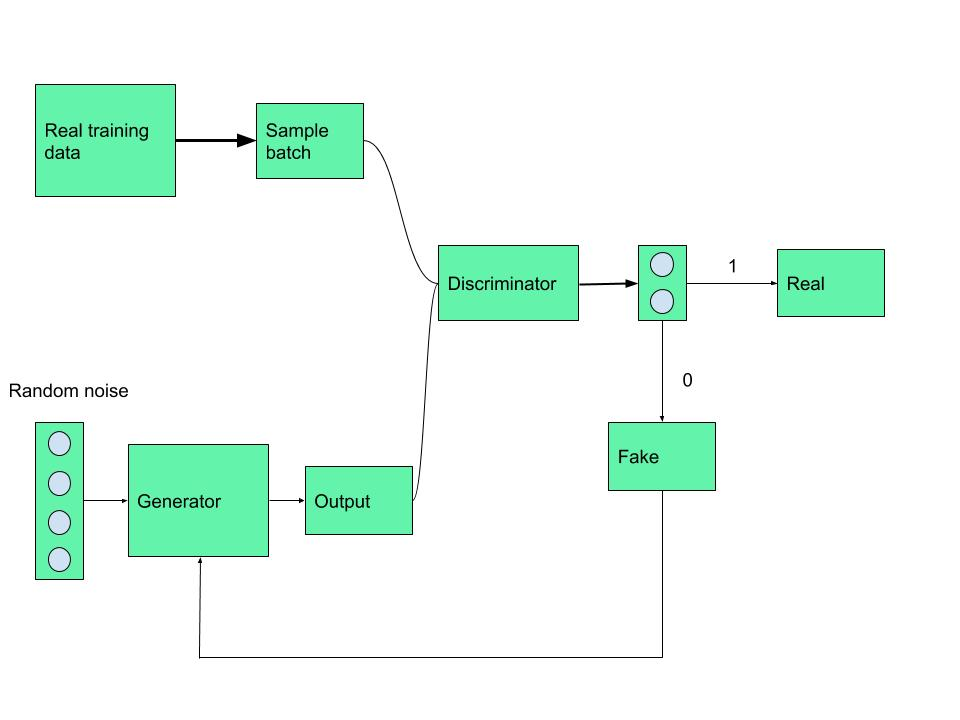# Generative Adversarial Networks (GANs) | An Introduction

• Difficulty Level : Easy
• Last Updated : 06 May, 2019

Generative Adversarial Networks (GANs) was first introduced by Ian Goodfellow in 2014. GANs are a powerful class of neural networks that are used for unsupervised learning. GANs can create anything whatever you feed to them, as it Learn-Generate-Improve.

To understand GANs first you must have little understanding of Convolutional Neural Networks. CNNs are trained to classify images with respect to their labels if an image is fed to a CNN, it analyzes the image pixel by pixel and is passed through nodes present in CNN’s hidden layers and as an output, it tells what the image is about or what it sees in the image.

For example:
If a CNN is trained to classify dogs and cats and an image is fed to this CNN, it can tell whether there is a dog or a cat in that image. Therefore it can also be called as a classification algorithm.

How GANs are different?

GANs can be divided into two parts which are the Generator and the Discriminator.

Discriminator –
This part of GANs can be considered similar to what CNNs does. Discriminator is a Convolutional Neural Network consisting of many hidden layers and one output layer, the major difference here is the output layer of GANs can have only two outputs, unlike CNNs, which can have outputs respect to the number of labels it is trained on.
The output of the discriminator can either be 1 or 0 because of a specifically chosen activation function for this task, if the output is 1 then the provided data is real and if the output is 0 then it refers to it as fake data.

Discriminator is trained on the real data so it learns to recognize how actual data looks like and what features should the data have to be classified as real.Generator –
From the name itself, we can understand that it’s a generative algorithm. Generator is an Inverse Convolutional Neural Net, it does exactly opposite of what a CNN does, because in CNN an actual image is given as an input and a classified label is expected as an output but in Generator, a random noise (a vector having some values to be precise) is given as an input to this Inverse CNN and an actual image is expected as an output. In simple terms, it generates data from a piece of data using its own imagination.As shown in the above image, a random value vector is given as input to Inverse-CNN and after getting passed through the hidden layers and activation functions an image is received as the output.

Working of both Generator and Discriminator together:
As we already discussed Discriminator is trained on actual data to classify whether given data is true or not, so Discriminator’s work is to tell what’s real and what’s fake.

Now the Generator starts to generate data from a random input and then that generated data is passed to Discriminator as input now Discriminator analyzes the data and checks how close it is to be classified as real, if the generated data does not contain enough features to be classified as real by the Discriminator, then this data and weights associated with it are sent back to the Generator using backpropagation, so that it can readjust the weights associated with the data and create new data which is better than the previous one. This freshly generated data is again passed to the Discriminator and it continues.
This process keeps repeating as long as the Discriminator keeps classifying the generated data as fakes, for every time data is classified as fake and with every backpropagation the quality of data keeps getting better and better and there comes a time when the Generator becomes so accurate that it becomes tough to distinguish between the real data and the data generated by the Generator.In Simple terms, Discriminator is a trained guy who can tell what’s real and what’s fake and Generator is trying to fool the Discriminator and make him believe that the generated data is real, with each unsuccessful attempt Generator learns and improves itself to produce data more real like. It can also be stated as a competition between Generator and Discriminator.

My Personal Notes arrow_drop_up